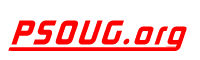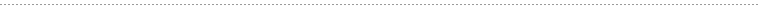Quick Search:PHP Code: Calculate File Size In Directory Jump to: Select a Location PSOUG Home Page Oracle Code Library mySQL Code Library PHP Code Library JavaScript Code Library Oracle Terms & Definitions Oracle Error Codes Advanced Code Search Contact UsSnippet Name: Calculate File Size In Directory

Description: This is a PHP Class that calculates the size, number of files & folders of a specific directory.

Comment: (none)

Language: PHP
Highlight Mode: PHP

```<?PHP

// calculate.directory.class.php
// Credits: BitRepository.com
// URL: http://www.bitrepository.com/web-programming/php/
// calculate-the-size-number-of-files-folders-of-a-directory.html

CLASS Directory_Calculator {

VAR \$size_in;
VAR \$decimals;

FUNCTION calculate_whole_directory(\$directory)
{
IF (\$handle = OPENDIR(\$directory))
{
\$size = 0;
\$folders = 0;
\$files = 0;

WHILE (FALSE !== (\$file = READDIR(\$handle)))
{
IF (\$file != "." && \$file != "..")
{
IF(IS_DIR(\$directory.\$file))
{
\$array = \$this->calculate_whole_directory(\$directory.\$file.'/');
\$size += \$array['size'];
\$files += \$array['files'];
\$folders += \$array['folders'];
}
ELSE
{
\$size += FILESIZE(\$directory.\$file);
\$files++;
}
}
}
CLOSEDIR(\$handle);
}

\$folders++;

RETURN ARRAY('size' => \$size, 'files' => \$files, 'folders' => \$folders);
}

FUNCTION size_calculator(\$size_in_bytes)
{
IF(\$this->size_in == 'B')
{
\$size = \$size_in_bytes;
}
ELSEIF(\$this->size_in == 'KB')
{
\$size = ((\$size_in_bytes / 1024));
}
ELSEIF(\$this->size_in == 'MB')
{
\$size = ((\$size_in_bytes / 1024) / 1024);
}
ELSEIF(\$this->size_in == 'GB')
{
\$size = ((\$size_in_bytes / 1024) / 1024) / 1024;
}

\$size = ROUND(\$size, \$this->decimals);

RETURN \$size;
}

FUNCTION size(\$directory)
{
\$array = \$this->calculate_whole_directory(\$directory);
\$bytes = \$array['size'];
\$size = \$this->size_calculator(\$bytes);
\$files = \$array['files'];
\$folders = \$array['folders'] - 1; // exclude the main folder

RETURN ARRAY('size'    => \$size,
'files'   => \$files,
'folders' => \$folders);
}
}
?>

Here&#39;s an usage example of this class:

example.php

<?PHP
SET_TIME_LIMIT(10000);

INCLUDE 'calculate.directory.class.php';

/* Path to Directory - IMPORTANT: with '/' at the end */

\$directory = '/home/mywebsite.com/public_html/';

/* Calculate size in: B (Bytes), KB (Kilobytes), MB (Megabytes), GB (Gigabytes) */

\$size_in = 'MB';

/* Number of decimals to show */

\$decimals = 2;

\$directory_size = NEW Directory_Calculator;

/* Initialize Class */

\$directory_size->size_in = \$size_in;
\$directory_size->decimals = \$decimals;

// return an array with: size, total files & folders
\$array = \$directory_size->size(\$directory);

ECHO "The directory <em>".\$directory."</em> has a size of
".\$array['size']." ".\$size_in.", ".\$array['files']." files &
".\$array['folders']." folders.";
?>```